Courses

# Work, Heat And Energy - Thermodynamic Chemistry Notes | EduRev

## Physical Chemistry

Created by: Asf Institute

## Chemistry : Work, Heat And Energy - Thermodynamic Chemistry Notes | EduRev

The document Work, Heat And Energy - Thermodynamic Chemistry Notes | EduRev is a part of the Chemistry Course Physical Chemistry.
All you need of Chemistry at this link: Chemistry

WORK, HEAT AND ENERGY

When process is isothermal: Ideal gas equation is: PV = nRT

When process is isothermal then

PV = constant
i.e., P1V1 = P2V2

According to first law dq = dU – ω
dq = dU + Pdv                                     (ω = –Pdv)
⇒ dq = CV dT + pdv                               (dU = CVdT)
When process is adiabatic then dq = 0

i.e. 0 = CVdT + PdV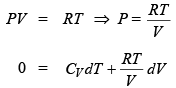⇒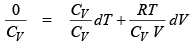⇒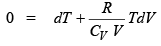⇒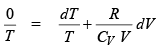⇒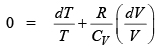⇒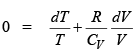putting the value o f CV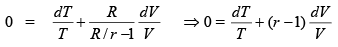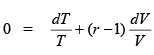Integrate this equation we get

Constant (C) = ln T + ln Vr – 1 = ln TVr – 1
i.e. TV r - 1 = constant

like this we can solve
PVr = constant,
PVr = constant
Vr – 1T = constant
TrP1 – r = constant

where γ is atomicity and is equal to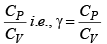Isothermal process: Is of two type (1) reversible and irreversible

ISOTHERMAL WORK, HEAT AND ENERGY

(A)  When process is reversible: We know that in reversible process infinit e small change takes place i.e. integration will be possible in reversible process.

Work in reversible process: We know that
W = - Pext dV

where P is pressure & dV is change in vo lume it is posit ive.
Let expansion of gas from volume V1 and V2 isothermal expansion.
W = – P dV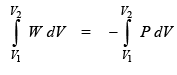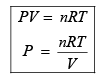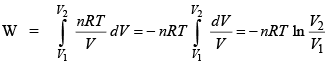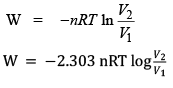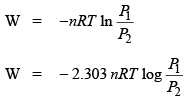where know in isothermal process

PV = constant
P1V= P2V2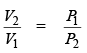When V1 < V2 or P1 > P2 then expansion takes place and work is negative.  When V1 > V2 or P1 < P2 then compression takes place & work is posit ive.

Energy in isothermal reversible process:
dH = nCPdT
when process is isothermal then dT = 0 then
dH = 0
dV = nCVdT

when process is isothermal then dT = 0 then
from first law, dq = dU – ω and
dU = 0 dq = –ω

Isothermal irreversible work, heat & energy: When process is irreversible then process is very fast and integration will not possible.

Work in irreversible process:

W = - Pext dV

Let expansion of gas from volume V1 and V2 from pressure Pto Pthen P2 is external pressure:

W = - Pext dV = –P2 (V2 – V1)
W = –P2 (V1 – V1)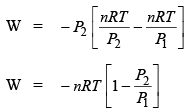P1V1 = nRT
P2V2 = nRT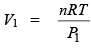and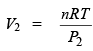If expansion of gas from volume V1 to V2 & P1 to Pagainst pressure P3 then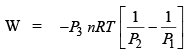Offer running on EduRev: Apply code STAYHOME200 to get INR 200 off on our premium plan EduRev Infinity!

,

,

,

,

,

,

,

,

,

,

,

,

,

,

,

,

,

,

,

,

,

,

,

,

;